Applications of Quadratics MathBitsNotebook.com Terms of Use   Contact Person: Donna RobertsLet's see how the Quadratic Formula can be applied in a variety of situations: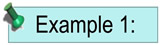The product of two positive consecutive even integer is 360. Find the numbers using a quadratic equation.
 Notice: this question asks for "positive" answers. Solution: Let x = first number x + 2 = second number x(x + 2) = 360 x2 + 2x - 360 = 0 (x + 20)(x - 18) = 0 x + 20 = 0; x = -20 (reject as the numbers are positive) x - 18 = 0; x = 18 (first number) x + 2 = 18 + 2 = 20 (second number) "Consecutive" means "one after another", such as: 12 and 13 are consecutive integers, 3 and 5 are consecutive odd integers, 8 and 10 are consecutive even integers.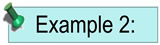One leg of a right triangle is seven more than the other leg. The hypotenuse is eight more than the shorter leg. Find the lengths of the three sides of the triangle.
When working with right triangles, think of the Pythagorean Theorem.
 Solution: Let x = short leg x + 7 = longer leg x + 8 = hypotenuse Apply the Pythagorean Theorem a2 + b2 = c2 x2 + (x + 7)2 = (x + 8)2 x2 + x2 + 14x + 49 = x2 + 16x + 64 2x2+ 14x + 49 = x2 + 16x + 64 x2 - 2x + 15 = 0 (x - 5)(x + 3) = 0 x - 5 = 0; x = 5 x + 3 = 0; x = -3 (reject as lengths are not negative) x = 5 x + 7 = 12 x + 8 = 13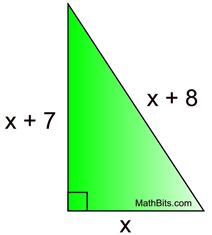Check in Pythagorean Theorem: 52 + 122 = 132 25 + 144 = 169 169 = 169 check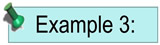The width of a rectangle is four less than its length. The area of the rectangle is 192 square units. Find the length and width of the rectangle.
 Pick out the key words in a problem. The word AREA stands out as a key word. Use algebraic expressions to represent the sides. Solution: Let x = the length x - 4 = the width Remember, the key word is AREA. Area = x(x - 4) = 192 x2 - 4x = 192 x2 - 4x - 192 =0 (either factor or use the quadratic formula) (x - 16)(x + 12) = 0 x - 16 = 0; x = 16 x + 12 = 0; x = -12 (length is not negative)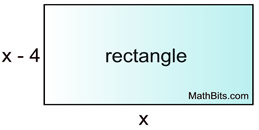Dimensions: 16 x 12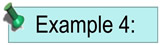A store's revenue from selling skateboards is determined by multiplying the number of skateboards by the cost per skateboard. The revenue, r, from selling x skateboards (where x is less than or equal to 50) is r = x(50 - 0.2x). How many skateboards need to be sold to have a revenue of \$480.
When a formula (equation) is given within the problem, use the formula.
The problem generally requires you to substitute values into the formula and solve for some value.
 Solution: Set the formula equal to \$480. 480 = x(50 - 0.2x) 480 = 50x - 0.2x2 0.2x2 - 50x + 480 = 0 (use quadratic formula)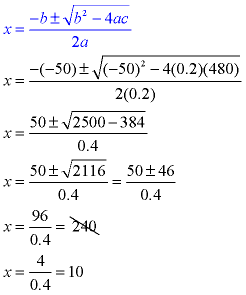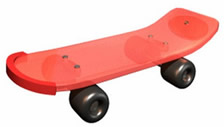Since the problem stated that x was less than or equal to 50, reject the solution of 240. They must sell 10 skateboards to have a revenue of \$480.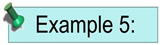The Allen family has installed a rectangular in-ground swimming pool. There is a concrete sidewalk around the pool with a consistent width of x feet. The outside edge of the sidewalk measures 24 feet by 41 feet. If the area of the pool is 630 square feet, find the width of the sidewalk.
Draw a diagram to get a better understanding of the situation.
 Solution: Since we know the "area" of the pool, let's start with area. The area of the pool can be expressed as: (24 - 2x)(41 - 2x) = 630 984 - 48x - 82x + 4x2 = 630 4x2 - 130x + 354 =0 (factor or quadratic formula) 2x2 - 65x + 177 = 0 (2x - 59)(x - 3) = 0 2x - 59 = 0; x = 29.5 ft (not realistic sidewalk width) x - 3 = 0; x = 3 feet (acceptable sidewalk width)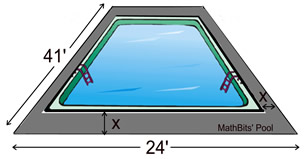[When determining the expressions to represent the sides of the pool, be sure to subtract the sidewalk width twice - once from each side of the pool. 24 - 2x and 41 - 2x ]